# Introduction

## tl;dr

• This solution got score of 4819 points on IBM Challenge Fall 2020 final excercise – what gives 2nd best score (preliminary).
• It is based on a permutation approach.
• Optimizations from the conceptual solution to the final circuit are described step by step.
• Complete code is presented.

## The IBM Challenge

The challenge was a three week long learning and competitive quantum programming event, hosted in November 2020, by IBM. The main theme was Programming for the Not-So-Distant Quantum Future. Like previous challenges it consisted of the educational part, where participants at various levels of quantum proficiency learned mainly about the Grover's algorithm, QRAM, and gate synthesis (at the Qiskit level). The last part was dedicated to implementation and optimization of Grover search for solving particular problem. Top ten participants were asked to prepare write-ups illustrating the approach and strategy taken to reduce the cost.

# The problem

## Plot

Dr. Ryoko is in danger of being stuck forever in one of the multiverses! However, she remains surprisingly calm given the threat that looms over her. I think he believes in our success more than we do! We will do our best to help her.

## Definition

We are given a 4×4 grid. 6 out of 16 cells are occupied by asteroids. The board is solvable when it is possible to choose three rows or colums, or a mix of them such that they will cover all the asteroids.

Now we are given 16 such boards and guaranteed that exactly one of them is unsolvable. Find that one (its id), using Lov Grover's algorithm with one iteration.

## Constraints

• The resulting circuit must use at most 28 qubits.
• The initial state for Grover's algorithm must be equal probability distributions.
• With the exception of the Unroller, which is required for decomposing your circuit to calculate quantum costs, you are not allowed to use any existing transpiler passes nor original transpilers for making simplifications.

## Cost function

• One-qubit gates cost 1.
• Two-qubit gate (CX) costs 10.

Optimize the circuit, providing the lowest score possible.

## Classical preprocessing

The purpose of this excercise is to make all the computations quantum-side, with no classical preprocessing of the data. This might sound quite vague so here is a list that summarizes restrictions imposed by the Jury:

• Oracle cannot require any knowledge of what the answers are. (For example, it is not allowed to create an oracle by using a classical optimization solver to get your answers for it.)
• When mapping the board information into quantum circuit, the board information must be unchanged. Do not rearrange the asteroid positions, nor rotate, invert, swap rows and columns.
• It is not allowed to create all the solvable boards (or unsolvable boards) classically, and then checking whether a given board matches to the solvable boards (or unsolvable boards). As a rule of thumb, the problem solving should be done by the quantum circuits, not classical subroutines.

## Example

Let us now examine a sample problem dataset.

We notice that all but one board are solvable using three shots:

# Solution

We utilize Kőnig's theorem, which can be summarized as follows.

If from the 6 occupied places we can choose 4, such that they don't share neither rows nor columns, the board cannot be solvable no matter how hard we try. See:

What happens in the opposite case? Imagine we can choose only 3 such asteroids. In terms of graph language and Kőnig's theorem, this set is called a (maximal) matching. The theorem actually provides a constructive proof that we can shoot all the asteriods within 3 shots. The steps are the following:

1. Mark all rows that are not present in the matching.
2. Repeat until no changes are made:
1. If in the current setting an asteroid from matching is shot, mark also its row for shooting.
2. If in the current setting an asteroid not from matching is shot, mark also its column for shooting.
3. Invert the choice of rows.

The following example illustrates steps of the algorithm:

And for yet another board, see the algorithm steps for two different initial matchings:

By the construction of the algorithm we observe the following:

1. During the algorithm, each column marking is followed by marking a row, keeping the number of selected rows+columns after the final inversion of rows choice constant (here: 3). In the case there is a marking of a column not followed by marking of a row, that implies that the initial matching provided was not maximal and tracing the rows/columns back gives us an augumenting path. That proves we get the required number of shots.
2. After the last iteration, each of element belonging to the matching is either shot by row and column, or none of them. Therefore, after inverting rows selection, each element from the matching is shot either by row or by column (not both). That proves that all the elements constituting the matching are shot.
3. After the last iteration, for each element not belonging to the matching the following holds. If it is row-shot, then it must be column-shot (by the construction of algorithm). Now by contraposition: if it is not column-shot, then it is not row-shot. After the final inversion we obtain that it is shot in both cases. That proves the remaining elements are shot as well.

More formal proof can be obtained by following Kőnig's theorem directly.

Combining the above observations we conclude that it is enough to check if there is a matching of size 4 to infer the board's unsolvability. In other words, we are going to check if there exists such permutation of columns that marked elements form a diagonal on the permuted board. And this is going to be the base of the proposed solution.

# Going quantum – sketch of the solution

In this challenge we are to implement the solution using celebrated Grover's algorithm with one iteration. Here is the sketch of the whole circuit conforming to this requirement.

• First we allocate some registers.
• As the first quantum operation we prepare an initial, equal superposition of our search space – 16 boards, using Hadamard transform on 4 qubits representing board ids.
• Next we apply an oracle, that flips the phase by $$\pi$$ radians of the states (boards) we look for.
• Then we use diffuser that effectively inverts the amplitude about the average of all the affected states. As a consequence it amplifies the states phased by an oracle earlier.
• After we are done with one Grover iteration, we proceed to measuring the results, hoping that the valid answer – unsolvable board – has the probability greater than the other elements.

The code is presented below. Next parts explain in details the whole implementation.

def solution_concept(problem_set):
boards = [read_board(board_spec) for board_spec in problem_set]

# Board id [0 - MSB, 3 - LSB]
qbid = QuantumRegister(4, name='bid')

# Buffer for the whole board (flattened, C memory order)
qbuf = QuantumRegister(16, name='buf')

# General-purpose ancillae
qanc = QuantumRegister(2, name='anc')

# Measured answer (endianness as in qbid)
cans = ClassicalRegister(4, name='c')

qc = QuantumCircuit(qbid,  qbuf, qanc, cans)

qc.h(qbid)
oracle_concept(qc, boards, qbid, qbuf, qanc)
diffuser_concept(qc, qbid, qanc)
qc.measure(qbid, cans)

return qc


## The oracle

This is the key part of the algoritm and the place where all the complexity comes from, as it must be designed to solve the particular problem. In our case it marks one of the board ids. Therefore we need to load the associated board data, mark state based on that data, and later unload the data, so that the phase change is the only effect of applying the oracle.

def oracle_concept(qc, boards, qbid, qbuf, qanc):
qram_concept(qc, boards, qbid, qanc, qbuf)
mark_unsolvable_concept(qc, qbuf, qanc)
qram_concept(qc, boards, qbid, qanc, qbuf)  # Unload the data / uncompute


def qram_concept(qc, boards, qbid, qanc, qbuf):
assert len(qbid) == 4
assert len(qbuf) == 16

obtain4_many_concept(
qc, boards,
[(r, c) for r in range(4) for c in range(4)], qbid, qbuf, qanc)


## Mark unsolvable

In this part, we assume having our data already in qbuf. The task, according to our solution is to detect if there is such permutation of columns for which occupied places form a diagonal, and this is equivalent to existence of matching of size 4. Here we just check all possible permutations and if we find a permutation being evidence of unsolvability of the board, we mark the state by applying Z rotation.

def mark_unsolvable_concept(qc, qbuf, qanc):
assert len(qbuf) == 16
assert len(qanc) >= 1

permwalk = permutations_walk_concept()
for pid, perm in enumerate(permwalk):
qc.ccx(qbuf[0*4 + perm], qbuf[1*4 + perm], qanc)
qc.ccx(qbuf[2*4 + perm], qbuf[3*4 + perm], qanc)
qc.cz(qanc, qanc)
qc.ccx(qbuf[2*4 + perm], qbuf[3*4 + perm], qanc)
qc.ccx(qbuf[0*4 + perm], qbuf[1*4 + perm], qanc)


For a moment we ignore the fact that there are boards with two such permutations, see images below. We will come back to this later.

We are going to walk over all the permutations in any order.

def permutations_walk_concept():
return list(permutations(range(4)))


## Diffuser

It is a final step, that utilizes phase difference we prepared earlier to amplify the differing state. Inversion about the average is another name for it. Here we present the simplest implementation.

def diffuser_concept(qc, qbits, qanc):
qc.h(qbits)
qc.x(qbits)
qc.append(ZGate().control(len(qbits) - 1), qbits)
qc.x(qbits)
qc.h(qbits)

qc = QuantumCircuit(4)
diffuser_concept(qc, Q[:4], [])
display_circuit(qc)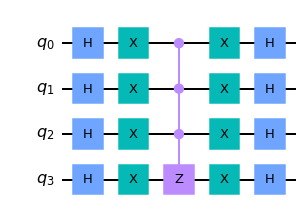## Obtainator

To complete the code we need to provide the subroutine for loading the data into quantum state. This is quite straightforward. Assuming we have qctrl bit marked for some board id (bid), we CX with that control qubit, all the qubits in the board buffer if (conditioned classically on the input data) there is a threat coming from an asteroid at that position.

def obtain4_many_concept(qc, boards, coords, qbid, qbuf, qanc):
def func(bid, qctrl):
for (r, c), qtarget in zip(coords, qbuf):
if boards[bid][r, c]:
qc.cx(qctrl, qtarget)
obtain4_walk_concept(qc, boards, func, qbid, qanc)


### Obtain walk

Again, the simplest possible solution for preparing the control ancilla.

def obtain4_walk_concept(qc, boards, func, qbid, qanc):
assert len(qanc) >= 1

for bid in range(16):
def x():
if bid & 0b0001 == 0: qc.x(qbid)
if bid & 0b0010 == 0: qc.x(qbid)
if bid & 0b0100 == 0: qc.x(qbid)
if bid & 0b1000 == 0: qc.x(qbid)

x()
qc.append(XGate().control(4), qbid[:] + [qanc])
func(bid, qanc)
qc.append(XGate().control(4), qbid[:] + [qanc])
x()


## Testing the solution

We have finalized the concept of the solution. Now it is the time to verify if the code works correctly.

simulate(solution_concept(problem_set), title='Concept of the solution')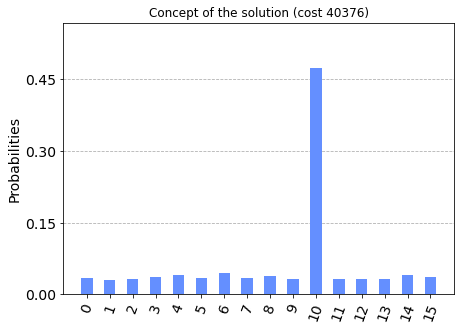As we see, the non-solvable board's amplitude was nicely amplified. The total cost of the solution is, however, far unsatisfying. So the next, more interesting part is dedicated to optimization of the implementation of this idea.

# Optimizing

## Margolus gate

As the solution is mainly based on the Boolean logic, one of the basic operations we apply in our circuit all the time is CCX (Toffoli) gate. Below we see its standard implementation, which uses 6 CX gates (this is the optimum).

qc_ccx = QuantumCircuit(3)
qc_ccx.ccx(0,1,2)
display_circuit(qc_ccx, unroll=True)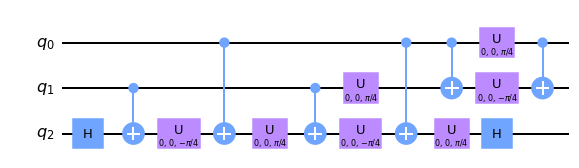That really costs a lot, making in fact CCX count in our circuit main contribution to the sum. However, there is a better alternative. Qiskit provides rccx – being relative CCX gate. Its cost is reduced to only 3 CX! But it has a major disadvantage: its relativeness means that it may (and actually does) change the phase of some states.

qc_rccx = QuantumCircuit(3)
qc_rccx.rccx(0,1,2)
display_circuit(qc_rccx, unroll=True)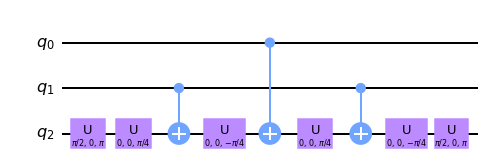Examining its unitary matrix, we can conclude that it is 2-nd root of unity, and control bits are not treated equally, i.e. rccx(a, b, c) != rccx(b, a, c). Because of that, we need to track carefully our rccx gate applications, especially whether the phase is properly uncomputed. For simple enough cases, however, it just works.

print_unitary(qc_rccx)

 1 0 0 0 0 0 0 0 0 1 0 0 0 0 0 0 0 0 1 0 0 0 0 0 0 0 0 1 0 0 0 0 0 0 0 0 1 0 0 0 0 0 0 0 0 -1 0 0 0 0 0 0 0 0 0 -i 0 0 0 0 0 0 i 0

As I'm more used to Margolus gate, further parts will be based on it. Margolus has very similar properties, being also the second root of unity. The effective unitary is different though – a bit simpler. The methods described later can be used in the same way regardless of rccx vs Margolus choice.

In the gate implementation, we introduce a concept of right_open and left_open to suppress emiting left and/or right one-qubit gates that might get cancelled when applying this gate to the same target.

def mrg(qc, q0, q1, q2, mask=(True, True),
left_open=False, right_open=False, right_cut=False):

if not left_open:
qc.ry(pi/4, q2)
qc.cx(q1, q2)

qc.ry(pi/4, q2)
else:
# qc.x(q2)
# qc.ry(pi/4, q2)
qc.u3(3/4*pi, pi, 0, q2)

qc.cx(q0, q2)

if not right_cut:

qc.ry(-pi/4, q2)
# qc.ry(-pi/4, q2)
# qc.x(q2)
qc.u3(3/4*pi, -pi, 0, q2)
# qc.x(q2)
# qc.ry(-pi/4, q2)
qc.u3(3/4*pi, 0, pi, q2)
# qc.x(q2)
# qc.ry(-pi/4, q2)
# qc.x(q2)
qc.u3(1/4*pi, 0, 0, q2)

qc.cx(q1, q2)

if not right_open:
qc.ry(-pi/4, q2)


The circuit implementation is very similar to rccx.

qc_mrg = QuantumCircuit(3)
mrg(qc_mrg, 0, 1, 2)
display_circuit(qc_mrg, unroll=True)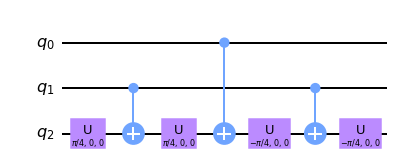It changes the phase of one state only.

print_unitary(qc_mrg)

 1 0 0 0 0 0 0 0 0 1 0 0 0 0 0 0 0 0 1 0 0 0 0 0 0 0 0 1 0 0 0 0 0 0 0 0 1 0 0 0 0 0 0 0 0 -1 0 0 0 0 0 0 0 0 0 1 0 0 0 0 0 0 1 0

The table summarizes the cost of single CCX-like gate call. The cost of rccx can be easily reduced to match Margolus, merging first and last pair of its one-qubit gates.

Cost
ccx69
rccx36
mrg34

### Anti-controlled version

Moreover, we also used the optimization for anti-controlled versions (parameter mask). A standard approach is to negate temporarily the control bit, which adds gates:

qc_mrg_x = QuantumCircuit(3)
qc_mrg_x.x(0)
mrg(qc_mrg_x, 0, 1, 2)
qc_mrg_x.x(0)
display_circuit(qc_mrg_x, unroll=True)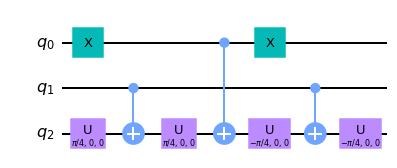Happily, this negation of a control bit can be succesfully moved to the target bit, merging with already present there one-qubit gate. Such implementation gives no extra overhead for anti-controlled versions of the gate. Here is an example with anti-control on the first bit:

qc_mrg_mask = QuantumCircuit(3)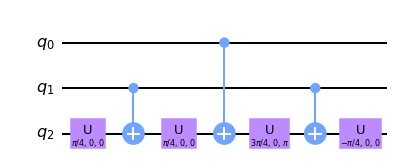Just to confirm two one-qubit gates saving:

Cost
qc_mrg_x36

### Common control and target

Yet another optimization comes from an observation of what happens if we apply two subsequent Margoluses sharing the target bit and the second control.

qc_mrg2 = QuantumCircuit(4)
mrg(qc_mrg2, 0, 2, 3)
mrg(qc_mrg2, 1, 2, 3)
display_circuit(qc_mrg2, unroll=True)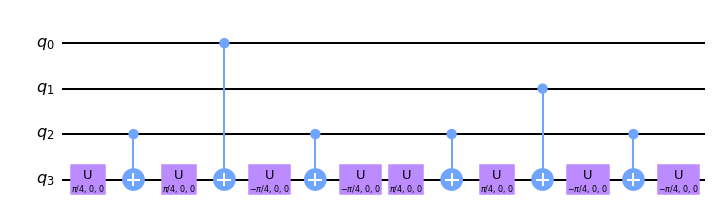Notice that a number of gates in the center of the circuit cancel-out, effectively reducing the count of CX gates. That motivates special implementation of mrg, allowing a sequence of first control bit.

def mrg_multi(qc, q0s, q1, q2,
left_open=False, right_open=False):
""" More general version of Margolus gate.
Applies mrg(q0, q1, q2), mrg(q0, q1, q2), ... """

if not left_open:
qc.ry(pi/4, q2)
qc.cx(q1, q2)
qc.ry(pi/4, q2)
for q0 in q0s:
qc.cx(q0, q2)
qc.ry(-pi/4, q2)
qc.cx(q1, q2)
if not right_open:
qc.ry(-pi/4, q2)


And here is the final result:

qc_mrg2_multi = QuantumCircuit(4)
mrg_multi(qc_mrg2_multi, (0, 1), 2, 3)
display_circuit(qc_mrg2_multi, unroll=True)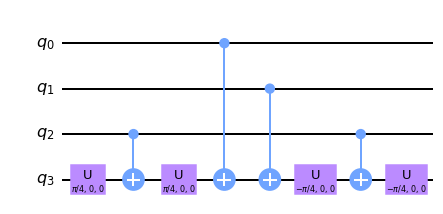Cost
mrg twice68
mrg_multi44

## Inversion realized in quantum

Let us now go back to the formulation of our solution using permutations. Each permutation has its parity – it corresponds to the parity of number of inversions that permutation is made of. Moreover, we can pair all the even permutations with odd ones. For instance we may propose such a pairing that after applying the $$(0 1)$$ inversion we obtain the second permutation from the pair. Proceeding according to this recipe we obtain the following two sets of permutations:

Even Odd
0123 1023
0231 1230
0312 1302
1320 0321
1203 0213

The trick we are going to perform is to split each original board into two boards – by introducing yet another Hadamard transoform. For the "odd" copy of the board, during QRAM loading part we will perform this inversion in the quantum state. It is enough to use 4 CSWAPs to swap items in two columns. Because of that, later in the oracle we can treat both copies the same way – using only even permutations for checks, as the $$(0 1)$$ inversion was conditionally preapplied to the second copy.

Effectively now, our search is not over 16 elements, but over 32 elements in total. Each permutation proving unsolvability of the board will be marked by a phase oracle with $$\pi$$ angle Pauli Z rotation. Amplification by the diffuser follows. Discarding, or even not measuring the added inversion bit, we get the id of the unsolvable board with greater probability than the others.

By the way, using this technique of splitting permutations into even and odd, we adressed here the problem mentioned earlier with two permutations certifying unsolvability of one board. One can easily prove that if there are two such permutations, these must be of different parity. And more precisely in this case, there is at most one inversion between them.

Here we present applying CSWAPs conditioned on the added qbidi qubit:

def inverse_concept(qc, qbuf, qbidi):
assert len(qbuf) == 16
assert len(qbidi) == 1

for r in range(4):
a = qbuf[r*4+0]
b = qbuf[r*4+1]

qc.cswap(qbidi, b, a)


Now we need to modify the permutations_walk function to include only the even half of all 24 permutations.

def permutations_walk():
return [
(0, 1, 2, 3),
(0, 2, 3, 1),
(0, 3, 1, 2),
(1, 3, 2, 0),
(1, 2, 0, 3),
(1, 0, 3, 2),
(2, 0, 1, 3),
(2, 3, 0, 1),
(2, 1, 3, 0),
(3, 1, 0, 2),
(3, 2, 1, 0),
(3, 0, 2, 1),
]


### CSWAP

A bit of optimization can be also applied to the inverse function, using Margolus gate instead of CCX (in default implemnetation of CSWAP).

def inverse(qc, qbuf, qbidi):
assert len(qbuf) == 16
assert len(qbidi) == 1

for r in range(4):
a = qbuf[r*4+0]
b = qbuf[r*4+1]

# cswap
qc.cx(b, a)
mrg(qc, qbidi, a, b)
qc.cx(b, a)


We can observe how it reduces the cost of one inverse call.

Cost
before356
after216

Finding a good QRAM loader required few hours of manual clicking and moving gates around (as automated tools were disallowed) in Quirk – an excellent tool by Craig Gidney.

Here is the final circuit.

It utilizes Gray codes sequence to walk over all board ids. But moreover, it turned out that using more than necessary number of ancillae, allowed such distribution of bit swap costs for which the total cost is lower.

Also these ancillae are not uncomputed after completing the pass (right_open) – these are specially allocated for this purpose, and we save the cost of uncomputing and recomputing them again during the QRAM unloading.

In the loading stage we will be going from board id 1111 all the way to 0111 (without performing the last bit change to go back to 1111). In the unloading phase we will be going in reverse (we recognize it by starting parameter).

The function below just encodes the circuit linked above.

def obtain4_walk(qc, boards, func, qbid, qanc,
starting=0b1111, left_open=False, right_open=False,
**kwargs):
assert starting in (0b1111, 0b0111)
assert len(qanc) >= 5

bid = starting
if not left_open:
assert bid == 0b1111
open_obtain4_walk(qc, qbid, qanc)

for i in range(len(boards)):
# Encode data for bid
func(bid, qanc)

# Gray-code walk over the board ids
if i < 16 - 1:
swapbit = 0  # 0 - LSB, 3 - MSB
if (i+1) & 0b0001 == 0: swapbit += 1
if (i+1) & 0b0011 == 0: swapbit += 1
if (i+1) & 0b0111 == 0: swapbit += 1

if swapbit == 0:
qc.cx(qanc, qanc)
elif swapbit == 1:
qc.cx(qanc, qanc)
mrg(qc, qbid, qanc, qanc, mask=(False, True))
elif swapbit == 2:
qc.cx(qbid, qanc)
qc.cx(qanc, qanc)
mrg(qc, qbid, qanc, qanc)
elif swapbit == 3:
qc.x(qbid)
qc.cx(qanc, qanc)
mrg(qc, qbid, qanc, qanc)
qc.x(qanc)
qc.cx(qbid, qanc)
qc.x(qanc)
qc.cx(qbid, qanc)

bid ^= (1 << swapbit)

assert bid == (0b1000 ^ starting)

if not right_open:
close_obtain4_walk(qc, qbid, qanc, **kwargs)

def open_obtain4_walk(qc, qbid, qanc):
mrg(qc, qbid, qbid, qanc)
mrg(qc, qanc, qbid, qanc)
mrg(qc, qbid, qbid, qanc, mask=(False, True))
mrg(qc, qbid, qbid, qanc, mask=(True, False))
mrg(qc, qbid, qanc, qanc)

def close_obtain4_walk(qc, qbid, qanc,
small_diffuser=False, last_minute_opt=False):
mrg(qc, qbid, qanc, qanc,
right_open=True, right_cut=last_minute_opt)
mrg(qc, qbid, qbid, qanc,
if not small_diffuser:
mrg(qc, qbid, qbid, qanc,
mrg(qc, qanc, qbid, qanc,
right_open=True, right_cut=small_diffuser)
mrg(qc, qbid, qbid, qanc,
right_open=True, right_cut=small_diffuser)


For the close_obtain4_walk let us ignore the additional flags for a while (this will be explained later).

Now let us examine the cost savings for the whole obtain4_many introduced by this optimization.

Cost
before16608
after1726

### Complete QRAM part

Collecting what we have just discussed.

def qram(qc, inv, boards, qbid, qbidi, qbidanc, qbuf, **kwargs):
assert len(qbid) == 4
assert len(qbuf) == 16

def obt(inv):
obtain4_many(
qc, boards,
[(r, c) for r in range(4) for c in range(4)],
qbid, qbuf, qbidanc,
left_open=inv, right_open=not inv,
starting=[0b1111, 0b0111][inv],
**kwargs
)

if not inv:
obt(False)
inverse(qc, qbuf, qbidi)
else:
inverse(qc, qbuf, qbidi)
obt(True)


Total savings (loading inv=False and unloading inv=True)

Cost
before33216
after3549

## Mark unsolvable

Here there are a few optimizations to make.

### Use Margolus extensively

First of all, we use a specific ordering of permutations in permutations_walk:

permutations_walk()

[(0, 1, 2, 3),
(0, 2, 3, 1),
(0, 3, 1, 2),
(1, 3, 2, 0),
(1, 2, 0, 3),
(1, 0, 3, 2),
(2, 0, 1, 3),
(2, 3, 0, 1),
(2, 1, 3, 0),
(3, 1, 0, 2),
(3, 2, 1, 0),
(3, 0, 2, 1)]

It was choosen in such a way that either first or the second element of the permutation is kept unchanged between two subsequent permutations (first pair). As a consequence, two remaining elements keep changing with no common elements (second pair). We can benefit from the behavior of the first pair. Examine the first transition from (0, 1, …) to (0, 2, …). We are CCX-ing the ancilla with the presence of asteroids at the first two positions.

Normally, we would first have to unload the first pair, and then load the second one:

qc_swap_pairs = QuantumCircuit(4)
qc_swap_pairs.ccx(0, 1, 3)
qc_swap_pairs.ccx(0, 2, 3)
display_circuit(qc_swap_pairs)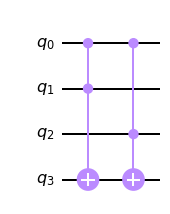But we recognize a pattern of two CCXes sharing one control bit, therefore we can apply mrg_multi to save extra CX gates.

### Delay T gates from CCZ

The next optimization comes from the decomposition of CCZ gate.

def ccz(qc, q0, q1, q2, open_=False):
if not open_:
qc.t(q2)
qc.t(q1)
qc.t(q0)

qc.cx(q0, q2); qc.tdg(q2);
qc.cx(q1, q2); qc.t(q2);
qc.cx(q0, q2); qc.tdg(q2);
qc.cx(q1, q2);
qc.cx(q0, q1); qc.tdg(q1); qc.cx(q0, q1)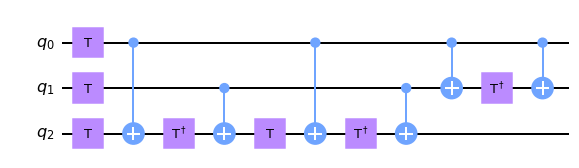CCZ gate is diagonal one.

 1 0 0 0 0 0 0 0 0 1 0 0 0 0 0 0 0 0 1 0 0 0 0 0 0 0 0 1 0 0 0 0 0 0 0 0 1 0 0 0 0 0 0 0 0 1 0 0 0 0 0 0 0 0 1 0 0 0 0 0 0 0 0 -1

Each of the three T gates opening the gate's decomposition is diagonal as well. This means that these all these parts commute with each other, allowing their reorganization. We will use this to delay execution of T gates. Two qubits involved in the CCZ will be input data, which is used only for these CCZs – therefore we can apply the needed one-qubit operation for qubits needing that, only once for all the permutations.

The third qubit is our working ancilla – used to mark the appearance of the first pair. We need to apply that T gate immediately – the best is to merge it with closing one-qubit gate of Margolus.

def close_mrg_and_t(qc, q):
# qc.ry(-pi/4, q)
# qc.t(q)
qc.u3(-pi/4, pi/4, 0, q)


This is the complete version of mark_unsolvable part.

def mark_unsolvable(qc, qbuf, qanc):
assert len(qbuf) == 16
assert len(qanc) >= 1

t_count =  * 16 # How many times to apply T gate to the buffer?

permwalk = permutations_walk()
for pid, perm in enumerate(permwalk):
if pid > 0:
prev = permwalk[pid-1]
if perm == prev:
mrg_multi(qc, (qbuf[1*4 + perm], qbuf[1*4 + prev]),
qbuf[0*4 + perm], qanc, right_open=True)
elif perm == prev:
mrg_multi(qc, (qbuf[0*4 + perm], qbuf[0*4 + prev]),
qbuf[1*4 + perm], qanc, right_open=True)
else:
assert False
else:
# Just load first pair for the first time
mrg(qc, qbuf[0*4 + perm], qbuf[1*4 + perm], qanc, right_open=True)

# Use second pair and qanc to perform action!
x = 2*4 + perm
y = 3*4 + perm

# CCZ parts
t_count[x] += 1
t_count[y] += 1
close_mrg_and_t(qc, qanc)

ccz(qc, qbuf[x], qbuf[y], qanc, open_=True)

if pid == 12 - 1:
mrg(qc, qbuf[0*4 + perm], qbuf[1*4 + perm], qanc)

# Apply the outstanding T gates
for i in range(16):
if t_count[i] % 8 != 0:
qc.u1(pi/4 * t_count[i], qbuf[i])


Halving the number of permutations and applying Margolus gates gives yet another score reduction of mark_unsolvable routine.

Cost
before6912
after1328

## Diffusion operator

Our search space containing $$2^5 = 32$$ states is spanned on $$5$$ qubits. Therefore to amplify the ones with altered phase, we need to construct 5-qubit diffuser. According to the original recipe, the diffusion operator is realized by the following circuit.

def diffusion5_concept(qc, qbits):
qc.h(qbits)
qc.x(qbits)
qc.append(ZGate().control(len(qbits) - 1), qbits)
qc.x(qbits)
qc.h(qbits)

qc = QuantumCircuit(5)
diffusion5_concept(qc, Q[:5])
display_circuit(qc)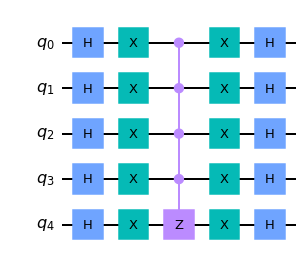The cost of CCCCZ is, however, quite high. Let us use two ancillae to lower the cost: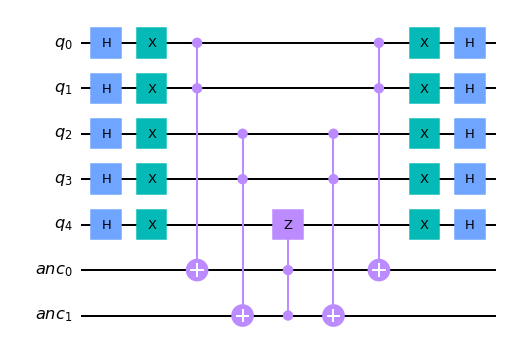Finally, let's calculate the product of H and X gate (and its inverse), used in the diffuser to merge a few one-qubit gates.

def hx(qc, q):
qc.u3(pi/2, 0, 0, q)

def xh(qc, q):
qc.u3(-pi/2, 0, 0, q)

def diffusion5(qc, qbits, qanc):
assert len(qbits) == 5
assert len(qanc) >= 2

hx(qc, qbits)

mrg(qc, qbits, qbits, qanc)
mrg(qc, qbits, qbits, qanc)
ccz(qc, qanc, qanc, qbits)
mrg(qc, qbits, qbits, qanc)
mrg(qc, qbits, qbits, qanc)

xh(qc, qbits)


That gives us the following cost reduction for 5-qubit diffuser.

Cost
before520
after213

### Small diffusion operator

It turns out, that such a big diffuser is not necessarily needed to get the amplification. One can use a smaller one. For more than one iteration of Grover it is quite tricky to arrange right sets of qubits used for diffusion. You can read about it more in our publication about introducing structure to expedite quantum search. In our case, limited to one iteration, we can just apply the smallest sensible diffuser, namely the 2-qubit one. The amplification will be performed in 4-element subspaces defined by all the remaining qubits' values.

def diffusion2(qc, qbits):
assert len(qbits) == 2

hx(qc, qbits)
qc.z(qbits) #HXH
qc.cx(qbits, qbits)
qc.z(qbits) #HXH
xh(qc, qbits)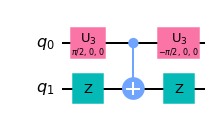Let us now summarize the cost savings for the diffuser.

Cost
concept (5q)520
optimized (5q)213
small (2q)14

The remaining decision to make is which of the qubits to use for diffusion in small diffuser setting. It strongly depends on the obtain4_walk implementation – especially the connections between board id qubits and the corresponding ancillae. We don't need to uncompute all the qubits used for obtaining data, those which don't interact with the diffuser have no impact on the measurement results. Our choice here is for 0th and 3rd qubit of the address.

We cannot choose the new qbidi qubit for small diffusion, as in case of two permutations marked per board, we would get no amplification for 2 out of 4 elements being marked.

def diffuser(qc, qbid, qbidi, qanc, small_diffuser):
assert len(qbid) == 4
assert len(qbidi) == 1

if small_diffuser:
diffusion2(qc, [qbid, qbid])
else:
diffusion5(qc, qbid[:] + [qbidi], qanc)


## Complete solution

The code that integrates all the parts of our optimized version.

def oracle(qc, boards, qbid, qbidi, qbidanc, qbuf, qanc, **kwargs):
qram(qc, False, boards, qbid, qbidi, qbidanc, qbuf, **kwargs)
mark_unsolvable(qc, qbuf, qanc)
qram(qc, True, boards, qbid, qbidi, qbidanc, qbuf, **kwargs)

def solution(problem_set, small_diffuser=False, last_minute_opt=False):
boards = [read_board(board_spec) for board_spec in problem_set]

# Board id [0 - MSB, 3 - LSB]
qbid = QuantumRegister(4, name='bid')

# Whether the inversion is applied
qbidi = QuantumRegister(1, name='bidi')

qbidanc = QuantumRegister(5, name='bidanc')

# Buffer for the whole board (flattened, C memory order)
qbuf = QuantumRegister(16, name='buf')

# General-purpose ancillae
qanc = QuantumRegister(2, name='anc')

# Measured answer (endianness as in qbid)
cans = ClassicalRegister(4, name='c')

qc = QuantumCircuit(
qbidi, qbid, qbidanc, qbuf,
qanc,
cans,
)

# Initial superposition
qc.h(qbid)
qc.h(qbidi)

oracle(qc, boards, qbid, qbidi, qbidanc, qbuf, qanc,
small_diffuser=small_diffuser,
last_minute_opt=last_minute_opt)

diffuser(qc, qbid, qbidi, qanc,
small_diffuser=small_diffuser)

qc.measure(qbid, cans)

return qc


## Optimization results

Here is the summary of the solutions submitted.

qc = solution(problem_set)
print('Operations:', qc.count_ops())
print('Cost:', compute_cost(qc))

Operations: OrderedDict([('cx', 482), ('ry', 165), ('tdg', 39), ('u3', 36), ('t', 16), ('u1', 8), ('x', 6), ('h', 5), ('measure', 4)])
Cost: 5095

qc = solution(problem_set, small_diffuser=True)
print('Operations:', qc.count_ops())
print('Cost:', compute_cost(qc))

Operations: OrderedDict([('cx', 459), ('ry', 145), ('tdg', 36), ('u3', 26), ('t', 12), ('u1', 8), ('x', 6), ('h', 5), ('measure', 4), ('z', 2)])
Cost: 4830


### Simulation

To confirm we didn't break anything.

simulate(solution(problem_set), title='Basic approach')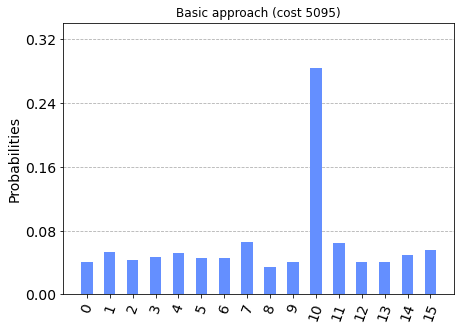simulate(
solution(problem_set, small_diffuser=True),
title='With small diffuser')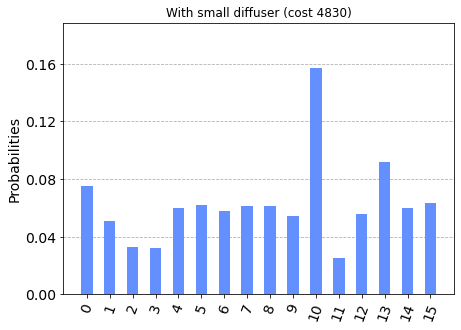In the histogram above we observe, that due to use of 2-qubit diffuser, the additional amplitude of board 10 (1010 binary) comes from boards 2, 3, 11 (0010, 0011, 1011 binary) – which is consistent with our expectations.

In the last hour of the contest I accidentally removed yet another gate. Since it worked for the example dataset, I made an extra submission. Given that I had no time to verify its correctess at that time, I left the final verdict to the Jury.

simulate(
solution(problem_set, small_diffuser=True, last_minute_opt=True),
title='With small diffuser and last-minute-opt')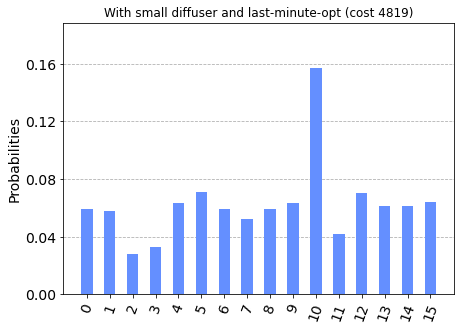# Closing words

This write-up presents the algorithm used for my contest submission. Further improvements are likely possible – both exploring further the optimizations mentioned, but also involving other techniques. For instance, the data transfer into quantum system could be a subject of compression. See Witek's write-up to see how he approached that. Even more ideas for optimization and different approaches could be found in the top 10 participants' write-ups.

At BEIT we do a lot like this, trying to get the most out of the currently available hardware – where each additional gate counts towards reduced circuit fidelity. Recently we published two papers on our experimental results on the unstructured search utilizing the mentioned before small diffusors technique: